# Assignment 3¶

We have information about several auctions at different times. The goal of the assignment is to predict whether a certain auction will be succesfull or not and then optimize a certain auction in order to get the most profit. The assignment consists of the following steps:

1) Descriptive Analysis
Here we do some basic exploratory analysis in order to get a feeling for the data and what we can do with it. We are allowed to also use correlation, plots and some simple inferences. Moreover, this is a nice way to start thinking about creating new features if neccesary.

2) Prediction Model
The goal is to build a prediction model that can either classify or predict the performance of a new auction. We are free to choose either classification or prediction as long as we are accurate since the model is used in step 3. Moreover, it should also be a relatively fast method in order to use it in our optimization in step 3.

3) Optimization
Given a new auction, optimize its features in such a way that you will maximize the final output. In other words, optimize the different variables and their values in a way to get the most money out of your auction. For example, if you have an item/lot that you expect will be worth 200 euros, then setting the starting bid at 50 euros or 100 euros might make a big difference on the end price. Each time we change a value, we check with our prediction model whether that change is a good one or not in order to eventually get the best "lay-out" of an auction.

Features
We have the following features in the dataset:

• Multiplier This is defined as (Winning Bid / Estimated Value)
• LotNr An items number, the lower the number the earlier the item is shown on the site
• Allocate Whether the seller of the lot has set a price which he want as minimum for the lot
• EstValue: estimated value of the lot (by auction experts)
• StartPrice: starting bidding price of the lot
• Followers: nr. of people following the lot
• Bank, Dealer, Liquidator, Volunteer: type of sales
• LotsSale: amount of lots of one sale
• LotsCtgry: amount of lots within a sale with the same category
• Forced: whether a sale is forced or not (due to bankruptcy)
• SP.EV: starting price/estimated value
• Duration: duration of auctions in hours on a lot
• Morning, Evening, Afternoon: last bid on the lot

### 1. Functions¶

In :
import math
import graphviz
import warnings
import random

import pandas                  as pd
import numpy                   as np
import seaborn                 as sns
import matplotlib.pyplot       as plt

from deap                      import base
from deap                      import creator
from deap                      import tools

from scipy.stats               import skew
from sklearn.tree              import DecisionTreeClassifier, export_graphviz
from sklearn.neighbors         import KNeighborsClassifier
from sklearn.svm               import SVC
from sklearn.ensemble          import RandomForestRegressor,VotingClassifier,AdaBoostClassifier, \
from sklearn.naive_bayes       import GaussianNB
from sklearn.linear_model      import LogisticRegression, Lasso, Ridge, ElasticNet
from sklearn.kernel_ridge      import KernelRidge

from xgboost                   import XGBClassifier, XGBRegressor
from catboost                  import CatBoostClassifier, CatBoostRegressor
from lightgbm                  import LGBMClassifier, LGBMRegressor

from sklearn.feature_selection import VarianceThreshold
from sklearn.model_selection   import StratifiedShuffleSplit, train_test_split, GridSearchCV,\
cross_val_score
from sklearn.metrics           import accuracy_score, log_loss, mean_squared_error
from sklearn.preprocessing     import StandardScaler, MinMaxScaler

from mlxtend.classifier        import StackingClassifier, StackingCVClassifier

%matplotlib inline
warnings.simplefilter("ignore", category=PendingDeprecationWarning) # LightGBM
warnings.simplefilter("ignore", category=DeprecationWarning)        # LightGBM
warnings.simplefilter("ignore", category=UserWarning)               # LightGBM

def preprocess_data(file_1_location = 'data2014.csv', file_2_location = 'data2015.csv'):

Parameters:
-----------
file_1_location (str): The location of the first csv file
file_2_location (str): The location of the first csv file

Returns:
--------
df (dataframe): Preprocessed dataframe

"""

# Load in Data

# Merge two files
df_2014['Year'] = 2014
df_2015['Year'] = 2015
df = df_2014.append(df_2015)
df = df.set_index(np.arange(len(df)))

# Extract part of day as a single variable and remove columns
df['Part_of_Day'] = df.apply(lambda row: get_part_of_day(row), axis = 1)
df['multiplier_binary'] = df.apply(lambda row: get_bin_multiplier(row['multiplier']), axis = 1)

df = df.drop(['Morning', 'Afternoon', 'Evening'], 1)

# Since we have the number of lots in a category, we can also say that each number of lots in a category
# is associated with a single category
# This is an assumption that might not hold, but it does give more information about having several categories
categories = {value: index+1 for index, value in enumerate(list(df['LotsCtgry'].unique()))}
df['Category'] = df.apply(lambda row: categories[row['LotsCtgry']], axis = 1)

# Test for consecutive rows
test_consecutive_sale(df)

# Create new column with sale number as the number of lots in a sale can be used as a proxy to which sale a lot belongs
sale_number = {value: index+1 for index, value in enumerate(list(df['LotsSale'].unique()))}
df['Sale_nr'] = df.apply(lambda row: sale_number[row['LotsSale']], axis = 1)

# # Get number of categories in a sale
df = get_categories_per_sale(df)

# Ordered lot numbers by category
ctgry_lot_nr_map = {(sales_nr,ctgry_nr,lot_nr): idx + 1
for (sales_nr,ctgry_nr),lot_nr_list
in df.groupby(['Sale_nr','Category']).LotNr.unique().items()
for idx, lot_nr in enumerate(lot_nr_list)}

df['CtgryLotNr'] = list(zip(*[df.Sale_nr,df.Category,df.LotNr]))
df['CtgryLotNr'] = df['CtgryLotNr'].map(ctgry_lot_nr_map)

# Binarize Year
year_map={2014:0,2015:1}
df.Year = df.Year.map(year_map)

# Create winning bid and the difference between winning bid and est. value
df['WinningBid'] = df.apply(lambda row: row['EstValue'] * row['multiplier'], axis = 1)
df['ResultDifference'] = df.apply(lambda row: row['EstValue'] * row['multiplier'] -
row['EstValue'], axis = 1)

# Create 3 classes
df['multiplier_three'] = df.apply(lambda row: three_classes(row), axis = 1)

return df

def get_part_of_day(row):
if row['Morning'] == 1:
return 0
elif row['Afternoon'] == 1:
return 1
elif row['Evening'] == 1:
return 2
else:
return 'Error!'

def get_bin_multiplier(row):
if row < 1:
return 0
elif row >= 1:
return 1
else:
return 'Error'

def test_consecutive_sale(df):
""" We want to know if the number of lots in a sale can be used
to indicate whether it belongs to a certain sale. This function
checks whether a consecutive series of LotsSale belong to each
other by keeping a set in which we store the LotsSale values
and check whether a new series of LotsSale has the same amount as
a series we have seen previously. If it doesn't return an error,
we can use the column "LotsSale" as a proxy for the lotnumber
"""
consecutive_check = set()
previous = ''

for i in df['LotsSale']:
if len(consecutive_check) == 0:
consecutive_check.update([i])
previous = i

if i == previous:
continue
else:
if i in consecutive_check:
print('Problem!')
break
else:
consecutive_check.update([i])
previous = i

def get_categories_per_sale(df):
""" Count for each sale how many categories there are in it
"""
test = df.groupby(by = ['Sale_nr', 'Category']).count().reset_index()
df['Categories_per_sale'] = 0

for i in df['Sale_nr'].unique():
df.loc[df['Sale_nr'] == i, 'Categories_per_sale'] = len(test[test['Sale_nr'] == i]['Category'].unique())

return df

def correlation_matrix(df):
""" Shows a correlation matrix

Parameters:
-----------
df (dataframe): Insert a dataframe with only the
columns that you want to visualize
"""
sns.set(style="white")

# Compute the correlation matrix
corr = df.corr()

# Generate a mask for the upper triangle
mask = np.zeros_like(corr, dtype=np.bool)

# Set up the matplotlib figure
f, ax = plt.subplots(figsize=(11, 9))

def scatter_plot(df, x, y, figsize = (10, 5), xlabel=None, ylabel=None, title=None):
""" Plots a scatterplot

Parameters:
-----------
df (dataframe): Data to be plotted
x (str): Name of column to be plotted
y (str): Name of column to be plotted
figsize (tup): Tuple of size
xlabel (str): Label x axis
ylabel (str): Label y axis
title (str): Title
"""

if not xlabel:
xlabel = x
if not ylabel:
ylabel = y
if not title:
title = x + ' vs. ' + y

plt.figure(figsize=figsize)
xseries = df[x]
yseries = df[y]
plt.xlabel(xlabel)
plt.ylabel(ylabel)
plt.title(title)
plt.plot(xseries,yseries, 'ro')
plt.show()

def multiple_scatter_plots(df, to_plot, figsize=(10, 5), markersize=2,  max_row=2):
""" Plots a scatterplot

Parameters:
-----------
df (dataframe): Data to be plotted
to_plot (list of tuples): Each tuples contains the column name of what you
want to plot on the x and y axis respectively
figsize (tup): Width and length of figures
marketsize (int): Size of dots
max_row (int): Number of plots per row

"""
# Initialize values and create figure
number_of_plots = len(to_plot)
max_column = math.ceil(number_of_plots / max_row)
fig, axs = plt.subplots(nrows=max_row, ncols=max_column, figsize=figsize)

# Flatten list if possible
try:
axs = [item for sublist in axs for item in sublist]
except:
pass

# Plot subplots
for i in range(len(to_plot)):
x = to_plot[i]
y = to_plot[i]
axs[i].plot(df[x], df[y], 'ro', markersize=markersize)
axs[i].set_xlabel(x)
axs[i].set_ylabel(y)
axs[i].set_title(x + ' vs. ' + y)

# Remove plots that are empty
empty_plots = abs(number_of_plots - (max_column * max_row))
if empty_plots > 0:
for i in range(1, empty_plots + 1, 1):
axs[-1].remove()

plt.show()

def plot_feature_importance(dataset, X, y):
""" Plot the importance of features in X

Parameters:
-----------
dataset (type): Dataset only containing relevant columns for X and y
X (numpy array): Matrix of features
y (numpy array): Target feature

"""

# Create random forest classifier
forest = RandomForestClassifier(n_estimators=250, random_state=42)
forest.fit(X, y)

# Calculate the relative importances of reatures
importances = forest.feature_importances_
std = np.std([tree.feature_importances_ for tree in forest.estimators_],axis=0)
indices = np.argsort(importances)[::-1]

# Plot the feature importances of the forest
plt.figure(figsize=(10, 4))
plt.title("Feature importances")
plt.bar(range(X.shape), importances[indices],
color="r", yerr=std[indices], align="center")
plt.xticks(range(X.shape), dataset.columns[indices], rotation=70)
plt.xlim([-1, X.shape])
plt.ylim([0, 0.4])
plt.show()

def plot_decision_tree(dataset, X, y, max_depth=3):
""" Plot decision tree to gain insight to feature importances

Parameters:
-----------
dataset (type): Dataset only containing relevant columns for X and y
X (numpy array): Matrix of features
y (numpy array): Target feature
max_depth (int): Depth of the decision tree

"""

dtree=DecisionTreeClassifier(max_depth=max_depth)
dtree.fit(X,y)

dot_data = export_graphviz(dtree, out_file=None,
feature_names=dataset.columns[:-1],
class_names=["0", "1"],
filled=True, rounded=True,
special_characters=True)
graph = graphviz.Source(dot_data)
return graph

def cv_multiple_classifiers(X, y, classifiers = [RandomForestClassifier()], n_splits = 5,
print_process = False):
"""

Parameters:
-----------
X (numpy array): Matrix of features
y (numpy array): Target feature
n_splits (int): Number of splits for cross validation

Returns:
--------
log (dataframe): Dataframe of the results

"""

# Dataframe to track results
log_cols = ["Classifier", "Accuracy"]
log = pd.DataFrame(columns=log_cols)
acc_dict = {name.__class__.__name__: 0 for name in classifiers}

# Method of cross validation
sss = StratifiedShuffleSplit(n_splits=n_splits, test_size=0.3, random_state=42)
fold = 1

# Cross-validation for each classifier
for train_index, test_index in sss.split(X, y):
if print_process:
print('Fold {}'.format(fold))
X_train, X_test = X[train_index], X[test_index]
y_train, y_test = y[train_index], y[test_index]

for clf in classifiers:
name = clf.__class__.__name__
clf.fit(X_train, y_train)
train_predictions = clf.predict(X_test)
acc = accuracy_score(y_test, train_predictions)
acc_dict[name] += acc

fold += 1

# Average out accuracies over number of splits
for clf in acc_dict:
acc_dict[clf] = acc_dict[clf] / n_splits
log_entry = pd.DataFrame([[clf, acc_dict[clf]]], columns=log_cols)
log = log.append(log_entry)

# Plot results
plt.xlabel('Accuracy')
plt.title('Classifier Accuracy')
sns.set_color_codes("muted")
sns.barplot(x='Accuracy', y='Classifier', data=log, color="b")

return log

def select_features(df, features = [], target = 'multiplier_binary'):
"""

Parameters:
-----------
df (dataframe): Dataframe that you want to select features from
features (list): List of the names (str) of the features you want
to extract as your X feature matrix
target (str): The column name of the feature that you want to extract

Returns:
--------
dataset (dataframe): Dataframe containig the features and target variables
X (numpy matrix): Numpy matrix of feature values
y (numpy array): Numpy array of the target values

"""

# Select a standard set of features if no other are given
if len(features) == 0:
features = ['LotNr', 'Allocate', 'EstValue', 'StartPrice','Followers', 'Bank', 'Dealer',
'Liquidator', 'Volunteer', 'LotsSale','LotsCtgry', 'Forced','SP.EV','Duration',
'Year', 'Category', 'Sale_nr', 'Categories_per_sale']
features.append(target)
dataset = df[features]

# Get X and y matrices
X = dataset.iloc[:, list(range(len(dataset.columns)-1))].values
y = dataset.iloc[:, -1].values

return dataset, X, y

def loo_cv(dataset, clf=RandomForestClassifier(n_estimators=100), adjust_folds=True):
""" Leave one-out cross validation

An implementation of leave one-out cross validation in which the folds
are determined by the sale number as we want to predict the multiplier of
sales we have not previously seen. Thereby creating an average accuracy score
that better represents reality. Folds in which the y_test shows very little
rows are removed as those are likely outliers.

Parameters:
-----------
dataset (dataframe):   Dataframe with only the features used
in the classification task including the target.
clf (classifier):      Classifier to be used for the Leave-one out
cross validation

Returns:
--------
results (list):      List of accuracies per fold
info_folds (dict):   Dictionary of lengths of y_test per fold with the intent
to remove folds that with less than 50 rows
"""

nr_folds = len(dataset['Sale_nr'].unique())
info_folds = {fold: '' for fold in range(1, nr_folds+1)}
results = []

for sale_nr in range(1, nr_folds+1):
X_train = dataset[dataset['Sale_nr']!= sale_nr].iloc[:,0:-1].values
X_test = dataset[dataset['Sale_nr']== sale_nr].iloc[:,0:-1].values

y_train = dataset[dataset['Sale_nr']!= sale_nr].iloc[:,-1].values
y_test = dataset[dataset['Sale_nr']== sale_nr].iloc[:,-1].values

name = clf.__class__.__name__
clf.fit(X_train, y_train)
train_predictions = clf.predict(X_test)
acc = accuracy_score(y_test, train_predictions)

results.append(acc)
info_folds[sale_nr] = len(y_test)

folds_to_remove = [fold-1 for fold in info_folds.items() if fold<50]
results = [value for index, value in enumerate(results) if index not in folds_to_remove]
for fold in folds_to_remove:
del info_folds[fold+1]

return results, info_folds

def three_classes(row):
""" Create 3 classes of the multiplier variable:
0 for multiplier < 1
1 for multiplier between 1 and 2
2 for multiplier larger than 2
"""
if row['multiplier'] < 1:
return 0
elif row['multiplier'] > 1.5:
return 2
else:
return 1

def plot_multiplier_distribution():
""" A quick plot to demonstrate outliers
in the data with respect to the variable
multipliers.
"""

plt.figure()
plt.subplot(121)
plt.hist(df['multiplier'], 60,  facecolor='g', alpha=0.75)
plt.xlabel('Multiplier')
plt.ylabel('Count')
plt.title('Histogram of Multiplier')
plt.grid(True)

plt.subplot(122)
plt.hist(df['multiplier'], 60,  facecolor='g', alpha=0.75)
plt.xlabel('Multiplier')
plt.ylabel('Count')
plt.title('Zoomed in Version')
plt.axis([0, 60, 0, 10])
plt.grid(True)

plt.show()

def resolve_skewedness(df):
""" Resolving the skewedness with features
that show a skew over .65 and normalizing
those features with a log(1+p) transform.

"""
# # Selecting only the numeric features
numeric_feature_names = df.dtypes[df.dtypes != "object"].index

# # Calculates the skewedness of the features and then gets the features with a skewedness above a certain threshold
skewed_features = df[numeric_feature_names].apply(lambda x: skew(x.dropna()))
skewed_features = list(skewed_features[skewed_features > 0.65].index)
skewed_features.remove('multiplier')
skewed_features.remove('multiplier_three')
skewed_features.remove('WinningBid')
skewed_features.remove('ResultDifference')

df[skewed_features] = np.log1p(df[skewed_features])

return df

def rmse_cross_validation(model, X, y):
""" Cross validation using RMSE as error measure
"""
rmse= np.sqrt(-cross_val_score(model, X, y, scoring="neg_mean_squared_error", cv = 5))
return(rmse)

def rmse(y_true, y_pred):
""" Calculate RMSE between predicted and true values
"""
return np.sqrt(mean_squared_error(y_true, y_pred))

def grid_search(param_grid, estimator):
""" Gridsearch implementation
"""
grid = GridSearchCV(estimator,param_grid,refit=True,verbose=2)
grid.fit(X, y)
print(grid.best_params_)
print(grid.best_estimator_)

def prepare_data_optimization(target = 'multiplier_binary'):
""" All preprocessing steps that are necessary for optimization

This function preprocesses the data, extract features, outlier removal,
feature engineering etc. Basically, it does all steps seen in this
notebook until the actual optimization. Thus, this also includes

Returns:
--------
df (dataframe): Preprocessed full data
to_optimize (dataframe): Only the data from sale_nr 10
which we want to optimize
baseline (int): The sum of all 1's in the to_optimize dataset
so we know what to beat.
X (numpy array): nD Array of features for training
y (numpy array): 1D Array of features for training

"""
# Preprocess data
df = preprocess_data()

# Remove outliers
df = df[df['multiplier'] <= 10]
df = df[df['EstValue'] < 15000]

# We choose to optimize sale_nr 10 as that sale has sufficient lots available to optimize
to_optimize = df.loc[df.Sale_nr == 10, :].copy()

if target == 'multiplier':
baseline = np.sum(to_optimize.multiplier.values * to_optimize.EstValue.values)
else:
baseline = np.sum(to_optimize.multiplier_binary)

# Initialize Decision variables
to_optimize.LotNr = range(1, len(to_optimize)+1, 1)
to_optimize.Duration = 50
to_optimize.Part_of_Day = 1
to_optimize.StartPrice = to_optimize.EstValue / 10

# Select only features for prediction, sale_nr is removed to prevent overfitting
features = ['LotNr', 'StartPrice','Duration','Part_of_Day','Allocate','EstValue','Followers',
'Bank','Dealer','Liquidator','Volunteer','LotsSale','LotsCtgry','Forced','Year',
'Category', 'Categories_per_sale','CtgryLotNr', 'RelStartPriceCtgry',
'RelDurationCtgry', 'RelLotNr','RelCtgryLotNr']
to_optimize = to_optimize[features]

# Create X and y for training the model by selecting all sales except sale_nr 10
df_train = df.loc[df.Sale_nr != 10, :].copy()
df_train = df[features + [target]]
dataset, X, y = select_features(df_train, features, target)

return df, to_optimize, baseline, X, y

def heuristic_probabilities(row, feature, interval):
""" Heuristic to optimize individual features

The heuristic optimizes the value of the feature variable
by iterating over values as defined by the interval. Thus,
a starting value is selected and added by the interval until
it improves. If after 10 iterations there has been no improvement,
it resets back to the starting value and goes the other direction.
For example, if adding 5 to the starting price of 40 doesn't yield
any improvement, reset back to the starting price of 40 and substract

Parameters:
-----------
row (numpy array): Array of features to optimize.
feature (string): The feature to optimize
interval (float): Intervals in which to optimize the feature

Returns:
--------
row (numpy array): Array of features that are optimized.

"""
best_probability = float(clf.predict_proba(row.reshape(1, -1)).flatten())
feature_index = to_optimize.columns.get_loc(feature) # Global, change this!
est_value = row[to_optimize.columns.get_loc('EstValue')]

start_row = row.copy()
temp_row = row.copy()

k = 0
count_consecutive = 0
list_probabilities = []

while k < 20:
temp_row[feature_index] += interval

# If feature to optimize is startprice, then it cannot be higher than EstValue
# In all other cases it only needs to be higher than zero as negative are impossible
if ((feature == 'StartPrice') and (temp_row[feature_index] < est_value)
and (temp_row[feature_index] > 0)):
new_probability = float(clf.predict_proba(temp_row.reshape(1, -1)).flatten())
elif (feature != 'StartPrice') and (temp_row[feature_index] > 0):
new_probability = float(clf.predict_proba(temp_row.reshape(1, -1)).flatten())
else:
return row, list_probabilities

# Changes direction of interval if there hasn't been improvement
# after 10 consecutive iterations.
if new_probability > best_probability:
row = temp_row.copy()
list_probabilities.append(new_probability - best_probability)
best_probability = new_probability
count_consecutive = 0
else:
count_consecutive += 1
if count_consecutive == 10:
interval = interval * -1
temp_row = start_row.copy()
k = 0
k += 1
return row, list_probabilities

def evalOneMax(individual, temp_to_optimize, target):
""" Evaluation Function to be used with DEAP.
It returns the sum of 1s for the target.
"""
for index, value in enumerate(individual):
temp_to_optimize.loc[index, target] = value
return np.sum(clf.predict(temp_to_optimize.values)),

def initialize_deap(to_optimize, target, min_val, max_val):
"""
Registrates the following:
attr_bool: Values (genes) taken between 0 and 300
individual: a set of genes that correspond to all starting prices for the dataframe to optimize
population: a list of individuals
evaluate: register the goal / fitness function
mate: the crossover operator
mutate:  a mutation operator with a probability to flip each attribute/gene of 0.05
select: selecting individuals for breeding next generation
"""
temp_to_optimize = to_optimize.copy()
temp_to_optimize = temp_to_optimize.reset_index(drop=True)

creator.create("FitnessMax", base.Fitness, weights=(1.0,))
creator.create("Individual", list, fitness=creator.FitnessMax)
toolbox = base.Toolbox()
toolbox.register("attr_bool", random.randint, min_val, max_val)
toolbox.register("individual", tools.initRepeat, creator.Individual, toolbox.attr_bool, len(to_optimize))
toolbox.register("population", tools.initRepeat, list, toolbox.individual)
toolbox.register("evaluate", evalOneMax, temp_to_optimize = temp_to_optimize, target = target)
toolbox.register("mate", tools.cxTwoPoint)
toolbox.register("mutate", tools.mutFlipBit, indpb=0.05)
toolbox.register("select", tools.selTournament, tournsize=3)

def heuristic_ga(to_optimize, target, min_val=0, max_val=300):
""" A genetic algorithm for optimization of the target.

Code was adopted from:
https://github.com/DEAP/deap/blob/master/examples/ga/onemax.py

Parameters:
-----------
to_optimize (dataframe): The dataframe to be optimized
target (str): The target column to optimize
min_val (float): Minimum value (>= 0) the target can take
max_val (float): Maximum value (> min_val) the target can take

Returns
to_optimize (dataframe): Dataframe with the target optimized
"""
random.seed(64)

# create an initial population of 300 individuals
toolbox, temp_to_optimize = initialize_deap(to_optimize, target, min_val, max_val)
pop = toolbox.population(n=300)

# CXPB is probability with which two individuals are crossed
# MUTPB is the probability for mutating an individual
CXPB, MUTPB = 0.5, 0.2

print("Start of evolution")

# Evaluate the entire population
fitnesses = list(map(toolbox.evaluate, pop))
for ind, fit in zip(pop, fitnesses):
ind.fitness.values = fit

print("  Evaluated %i individuals" % len(pop))

# Extracting all the fitnesses of
fits = [ind.fitness.values for ind in pop]

# Variable keeping track of the number of generations
g = 0

# Begin the evolution
while max(fits) < 100 and g < 1000:
g = g + 1
print("-- Generation %i --" % g)

# Select and clone the next generation individuals
offspring = toolbox.select(pop, len(pop))
offspring = list(map(toolbox.clone, offspring))

# Apply crossover and mutation on the offspring
for child1, child2 in zip(offspring[::2], offspring[1::2]):

# cross two individuals with probability CXPB
if random.random() < CXPB:
toolbox.mate(child1, child2)

# fitness values of the children
# must be recalculated later
del child1.fitness.values
del child2.fitness.values

for mutant in offspring:

# mutate an individual with probability MUTPB
if random.random() < MUTPB:
toolbox.mutate(mutant)
del mutant.fitness.values

# Evaluate the individuals with an invalid fitness
invalid_ind = [ind for ind in offspring if not ind.fitness.valid]
fitnesses = map(toolbox.evaluate, invalid_ind)
for ind, fit in zip(invalid_ind, fitnesses):
ind.fitness.values = fit

print("  Evaluated %i individuals" % len(invalid_ind))

# The population is entirely replaced by the offspring
pop[:] = offspring

# Gather all the fitnesses in one list and print the stats
fits = [ind.fitness.values for ind in pop]

length = len(pop)
mean = sum(fits) / length
sum2 = sum(x*x for x in fits)
std = abs(sum2 / length - mean**2)**0.5

print("  Min %s" % min(fits))
print("  Max %s" % max(fits))
print("  Avg %s" % mean)
print("  Std %s" % std)

print("-- End of (successful) evolution --")

best_ind = tools.selBest(pop, 1)
temp_to_optimize.loc[:, target] = best_ind
return temp_to_optimize

def print_results(data, clf):
""" Print final result of heuristics

It prints both the sum of 1s as well as the sum of
belonging to the first class.

Parameters:
-----------
data (list of tuples): Each tuple indicates the df with the corresponding name
E.g. results(data = [(df, 'Heuristic'), (df2, 'Heuristic 2'), clf])
clf (classifier): Classifier to be used for calculating results

"""
for df, name in data:
print(name)
sum_1s = np.sum(clf.predict(df.values))
sum_probabilities = np.sum([x if x > 0.5 else 0 for x in clf.predict_proba(df.values)])
print('Sum of 1s: {}'.format(sum_1s))
print('Sum of probs that class is 1: {}'.format(sum_probabilities))
print()

def validate_heuristic_results(clf, df, baseline, percentage=30, iterations=100):
""" Validation of results after using heuristic

The issue with using a classifier is that it is only
accurate for a certain percentage of items. For that
reason optimizing might be an issue. For example,
if a model is only accurate for 80% of a binary predictor,
then 80% of the optimized values are likely to be correct
and 20% likely incorrect. This function reverts x% of the
target (e.g. 1 to 0 and 0 to 1) as a way to simulate having
x% of the target classified incorrectly. Then the baseline
is again calculated. This is average over a set amount of
instances/iterations.

Parameters:
-----------
clf (model): Model that is used for prediction
df (dataframe): Dataframe that is optimized
baseline (int): Baseline with which to compare (sum of 1s)
percentage (float): percentage to be swapped
iterations (int): Number of iterations to average over
"""

temp = list(clf.predict(df.values))
indices = [np.random.choice(range(len(temp)), int(len(temp)/100*percentage),
replace=False) for i in range(iterations)]
multiple_tests = []

for sample in indices:
# Predict scores and invert them according to the sample
result = list(clf.predict(df.values))
for index in sample:
result[index] = (result[index] * -1) + 1

multiple_tests.append(np.sum(result))

print('Baseline: \t{}'.format(baseline))
print('Optimization: \t{}'.format(np.mean(multiple_tests)))

In :
%%html
<style>
table {float:left}
</style>


### 2. Preprocess Data¶

Steps taken:

• Load and merge the 2014 and 2015 files
• Extract part of day as a single variable and remove columns
• Create a binary feature of the multipler variable
• Create category based on the number of lots per category (those are unique and therefore represent a category)
• Create Sale number based on the number of lost per sale (those are unique and therefore represent a lot)
• Create categories per sale
In :
df = preprocess_data()


Below you can see the data as was given to me without additional preprocessing of the data. The main purpose is to optimize the feature "multiplier" by finding optimal values for the decision variables.

In :
df[df.columns[:-10]].head()

Out:
multiplier LotNr Allocate EstValue StartPrice Followers Bank Dealer Liquidator Volunteer LotsSale LotsCtgry Forced SP.EV Duration Year Part_of_Day multiplier_binary Category
0 1.300000 6 0 700 400 19 0 1 0 0 1177 436 0 0.571429 67 0 2 1 1
1 0.616667 7 0 600 350 12 0 1 0 0 1177 436 0 0.583333 67 0 2 0 1
2 0.600000 9 0 1500 600 19 0 1 0 0 1177 436 0 0.400000 67 0 2 0 1
3 0.833333 10 0 300 150 20 0 1 0 0 1177 436 0 0.500000 67 0 2 0 1
4 0.600000 11 0 500 300 9 0 1 0 0 1177 436 0 0.600000 68 0 2 0 1

Newly created features
Several new features were created based on the initial set of features:

Feature Description
Multiplier_binary The multiplier variable in binary format (0 if value < 1 and 1 if value >= 1)
Category The number of the category for a certain item
Sale_nr The number of the sale
Categories_per_sale The number of categories per sale
CtgryLotNr Ordering of Lots within a category
RelStartPriceCtgry Relative starting price with regards to the category
RelDurationCtgry Relative duration with regards to the category
RelLotNr LotNr divided by LotsSale
RelCtrgyLotNr Relative amount of lots per category (CtgryLotNr / LotsCtgry)
WinningBid The winning bid (EstValue * multiplier)
ResultsDifference Difference between winning bid and est. value

### 3. Exploration¶

We have no missing values that we have to worry about.

In :
df.isnull().values.any()

Out:
False

It turns out there is a lot of data missing. The number of lots in sale indicate how many lots there should be in a sale.
However, when you actually count the number of lots, there seem to be some missing.

In :
for i in df['LotsSale'].unique():
nr_lots_suggested = i
nr_lots_actual = len(df[df['LotsSale'] == i])
print('Lots Expected: {}, \tLots in df: {},   \t Lots missing: {}'.format(nr_lots_suggested, nr_lots_actual,
nr_lots_suggested - nr_lots_actual))

Lots Expected: 1177, 	Lots in df: 783,   	 Lots missing: 394
Lots Expected: 1411, 	Lots in df: 979,   	 Lots missing: 432
Lots Expected: 95, 	Lots in df: 89,   	 Lots missing: 6
Lots Expected: 1223, 	Lots in df: 765,   	 Lots missing: 458
Lots Expected: 355, 	Lots in df: 36,   	 Lots missing: 319
Lots Expected: 905, 	Lots in df: 767,   	 Lots missing: 138
Lots Expected: 219, 	Lots in df: 157,   	 Lots missing: 62
Lots Expected: 295, 	Lots in df: 226,   	 Lots missing: 69
Lots Expected: 955, 	Lots in df: 730,   	 Lots missing: 225
Lots Expected: 422, 	Lots in df: 378,   	 Lots missing: 44
Lots Expected: 460, 	Lots in df: 243,   	 Lots missing: 217
Lots Expected: 191, 	Lots in df: 158,   	 Lots missing: 33
Lots Expected: 68, 	Lots in df: 34,   	 Lots missing: 34
Lots Expected: 17, 	Lots in df: 14,   	 Lots missing: 3
Lots Expected: 1426, 	Lots in df: 1152,   	 Lots missing: 274
Lots Expected: 462, 	Lots in df: 390,   	 Lots missing: 72
Lots Expected: 646, 	Lots in df: 507,   	 Lots missing: 139
Lots Expected: 1053, 	Lots in df: 877,   	 Lots missing: 176
Lots Expected: 721, 	Lots in df: 526,   	 Lots missing: 195
Lots Expected: 1329, 	Lots in df: 996,   	 Lots missing: 333
Lots Expected: 89, 	Lots in df: 62,   	 Lots missing: 27
Lots Expected: 1079, 	Lots in df: 771,   	 Lots missing: 308
Lots Expected: 161, 	Lots in df: 101,   	 Lots missing: 60
Lots Expected: 291, 	Lots in df: 3,   	 Lots missing: 288
Lots Expected: 326, 	Lots in df: 252,   	 Lots missing: 74
Lots Expected: 280, 	Lots in df: 201,   	 Lots missing: 79
Lots Expected: 162, 	Lots in df: 119,   	 Lots missing: 43


Most likely the data that is missing are lots that couldn't be sold as nobody would place a bid.

### 4. Visualizations¶

#### 4.1 Plots¶

After some playing around, I found the following (possibly) interesting plots to show some form of relationship,
at least to the human eye. Further exploration seems to be neccesary with regards to the Estimated value
which is why next you can see the correlations.

In :
multiple_scatter_plots(df, [('EstValue', 'multiplier'),('StartPrice', 'multiplier'),
('Followers', 'multiplier'),('Followers', 'LotNr')], figsize=(10, 8),
max_row=2)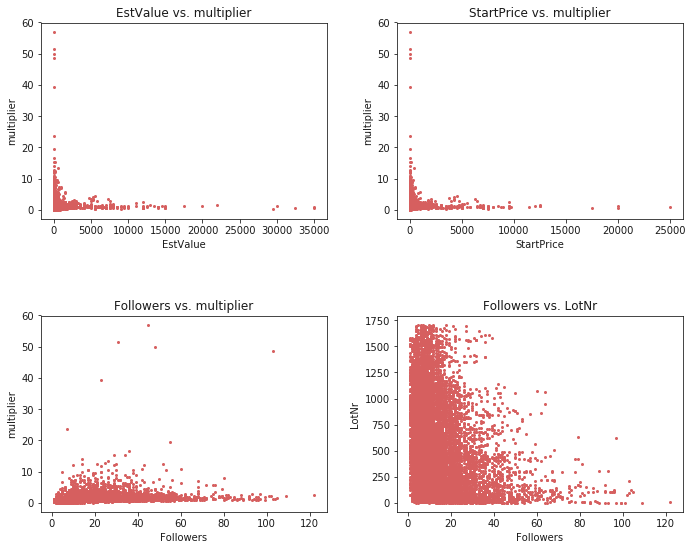In :
scatter_plot(df, 'EstValue', 'StartPrice')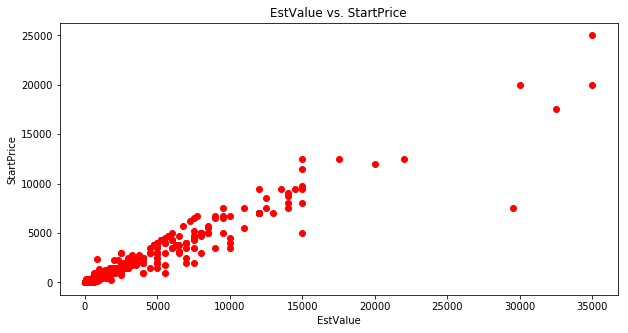In :
plot_multiplier_distribution()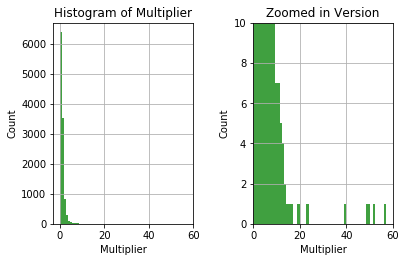#### 4.2 Correlations¶

This is where things get really interesting. Why? Because, unfortunately, there is a high correlation between the Estimated Value and the Start Price.
This basically indicates that the Starting Prices are likely to be a standard percentage of the Estimated Value.
In other words, we cannot infer how changing the Starting Price would affect the multiplier as it is always the same, which is a problem

In :
correlation_matrix(df)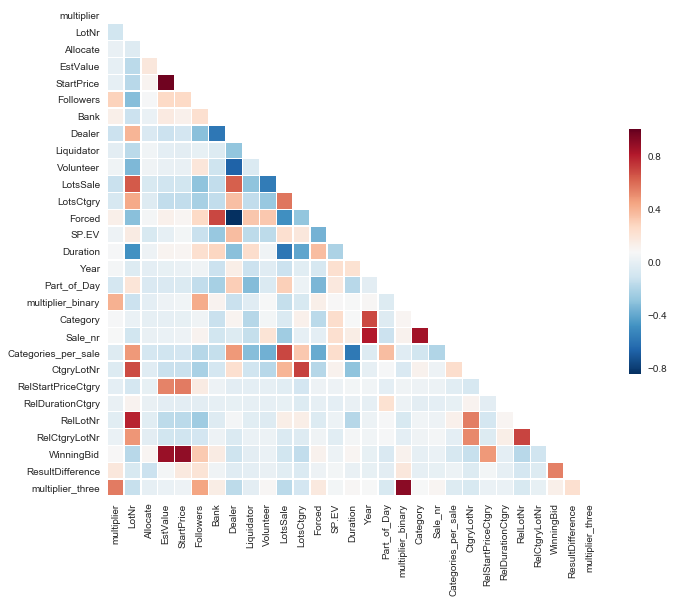In :
corr_matrix = df.corr().abs()
corr_matrix = corr_matrix.where(np.triu(np.ones(corr_matrix.shape),
k=1).astype(np.bool)).stack().sort_values(ascending=False)
corr_matrix[:10]

Out:
EstValue           StartPrice          0.972891
multiplier_binary  multiplier_three    0.909424
StartPrice         WinningBid          0.899354
EstValue           WinningBid          0.871405
Category           Sale_nr             0.852410
Dealer             Forced              0.839268
Year               Sale_nr             0.818900
LotNr              RelLotNr            0.788180
LotsCtgry          CtgryLotNr          0.711148
RelLotNr           RelCtgryLotNr       0.704299
dtype: float64

### 5. Feature Engineering¶

#### 5.1 Outlier Removal¶

As seen before it looks like the target variable 'multiplier' shows some outliers for the multiplier with a value higher than 10. Especially regressors are highly affected by outliers and decrease the resulting score. Therefore, we remove the outliers in this sample. It should be noted that there was no possibility to discuss with the owners of the data as domain expertise would have helped. They could have helped in advising whether to remove the outliers or not and which points to regard as outliers.

In :
outlier_percentage = len(df[df['multiplier']>10]) / len(df) * 100
print('{} % of outliers with regards to the multiplier'\
.format(round(outlier_percentage,2)))
df = df[df['multiplier'] <= 10]

0.26 % of outliers with regards to the multiplier

In :
outlier_percentage = len(df[df['EstValue']>=15000]) / len(df) * 100
print('{} % of outliers with regards to the EstValue'\
.format(round(outlier_percentage,2)))
df = df[df['EstValue'] < 15000]

0.12 % of outliers with regards to the EstValue


Thus, we can clearly see that we removed 0.26% of the outliers when removing all rows that are associated with a multiplier score of higher than 10.

#### 5.2 Skewedness¶

In order to get a good score out of you regressors you often have to fix a skewed distribution of your feature variables. Regressors in most cases expect a normal distribution that has no skew. Therefore, we would like to do a log(1+p) transform for the variables that show a skew of larger than 0.65. It should be noted though that we only do this for the regression later on as this will not have any effect on

In :
# df = resolve_skewedness(df)


### 6. Feature Importance¶

Here we are going to look into the importance of some features.
Preferably, we would like to see that important features are those that can be selected as decision variables.

#### 6.1 Decision Tree¶

A decision tree is used to give a quick and intuitive feeling on what features are important.

In :
features = ['LotNr','Allocate','EstValue','StartPrice','Followers','Bank','Dealer','Liquidator','Volunteer','LotsSale',
'LotsCtgry','Forced','SP.EV','Duration','Year','Category','Sale_nr','Categories_per_sale','CtgryLotNr',
'RelStartPriceCtgry', 'RelLotNr', 'RelCtgryLotNr','RelDurationCtgry']
target = 'multiplier_binary'
dataset, X, y = select_features(df, target = target)
plot_decision_tree(dataset, X, y, max_depth=3)

Out:

#### 6.2 Random Forest¶

A random forest is a better way of indicating which features are important as it can see for each tree in the forest which features allow for the highest increase/decrease in gini/entropy. Unfortunately, Followers is highest and clearly not a decision variable as those are decided beforehand.
However, LotNr seems to be an importance feature and is something we can definitely optimize!

In :
plot_feature_importance(dataset, X, y)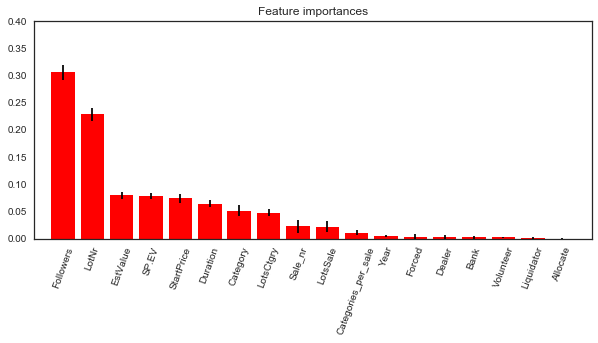#### 6.3 Feature Selection¶

This an important part of most parts that will follow. This piece of code will let you quickly select
the feature variables that you want in your "X" variable and the target feature variable in "y".
Moreover, a dataframe called "dataset" will be generated that includes the features and target variables.
The target variable will always be the last column in dataframe "dataset".

You can use this code to change the variables in the Predictions, GridSearch and Feature Importance.

In :
features = ['LotNr','Allocate','EstValue','StartPrice','Followers','Bank','Dealer',
'Liquidator','Volunteer','LotsSale','LotsCtgry','Forced','SP.EV',
'Duration','Year','Category','Sale_nr','Categories_per_sale','CtgryLotNr',
'RelStartPriceCtgry', 'RelLotNr', 'RelCtgryLotNr','RelDurationCtgry']
target = 'multiplier_binary'
dataset, X, y = select_features(df, features, target)


### 7. Prediction¶

#### 7.1 Classification with K-Fold¶

The initial results show that a Random Forest performs best, even with no parameters tweaked. Some tweaking is ofcourse necessary in order to get the best results.
It should be noted though that I haven't thought yet about the best way to do cross validation in this case. For now, it is simply 5-fold cross validation.
A thought would be to use leave-one out as we have multiple different sales and we can see how it would perform if we would predict for each sale.
It would definitely most relevant to the optimization as we are (probably) going to optimize individual sales.

In :
clf = [KNeighborsClassifier(), LGBMClassifier(),DecisionTreeClassifier(),
GaussianNB(),LogisticRegression(),XGBClassifier()]
result = cv_multiple_classifiers(X, y, classifiers = clf, n_splits=10, print_process=False)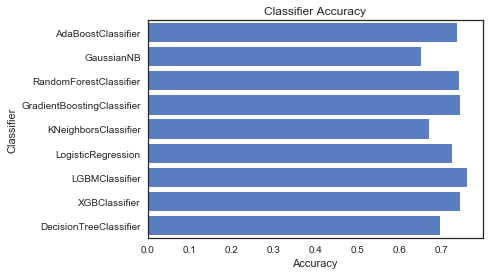#### 7.2 Classification with Leave-One-Out CV¶

The issue with normal k-fold cross validation is that there will be leakage of data with respect to individual sales. The purpose of cross validation is to prove that your model works on data that it has not seen before. However, if you use k-fold cross validation then it it likely that information regarding a certain sale will end up in both the train and test sets. Therefore, we adopted leave-one-out (LOO) cross validation which is essentially a k-fold cross validation where the k's are defined by the sales themselves. Seeing as we have 27 sales in the dataset, LOO CV will give us a 27-fold cross validation which is a more accurate than any normal k-fold CV.

In :
rfc = RandomForestClassifier(n_estimators=100, max_features= 11)
lgbm = LGBMClassifier(learning_rate=0.2, max_depth=2,metric='binary_logloss', min_child_samples=20,min_data_in_leaf=15, num_leaves=2, objective='binary',random_state=42)
xgb = XGBClassifier(gamma=1, learning_rate=0.000001,max_depth=1, min_child_weight=1,n_estimators=100,n_jobs=-1, objective='binary:logistic', random_state=42,reg_alpha=0.5, reg_lambda=0.5)
results, info_folds = loo_cv(dataset, adjust_folds=False, clf=lgbm)


27 folds/sales in the cross validation is fairly large, which is typically not an issue if each fold has enough data. However, there are some folds/sale that only have a few lots in them. What you then get is that you have 99% of the data is trained and only 1% is tested on. When you test on 1% of the data, then it is likely you get outliers, either very high or very low accuracy scores. Therefore, I simply removed the accuracy of folds where the target fold has 50 or less datapoints.

In :
folds_to_remove = [fold-1 for fold in info_folds.items() if fold<50]
results_new = [value for index, value in enumerate(results) if index not in folds_to_remove]
print('old: {}'.format(sum(results)/len(results)))
print('new: {}'.format(sum(results_new)/len(results_new)))

old: 0.7083953661270547
new: 0.718611468448668


#### 7.3 Voting Classifier with Leave-One-Out CV¶

Instead of choosing a single classifier, let's use multiple classifiers and see if they agree on a better model than simply using a single one. The idea is simple, all classifiers make mistakes but they don't all make the same mistakes. It's a principle very similar to Random Forests and ensembles in general.

In :
clf1 = RandomForestClassifier(n_estimators=100, max_features= 11, random_state=42)
clf4 = LGBMClassifier(learning_rate=0.2, max_depth=2,metric='binary_logloss', min_child_samples=20,min_data_in_leaf=15, num_leaves=2, objective='binary',random_state=42)
clf5 = XGBClassifier(gamma=1, learning_rate=0.000001,max_depth=1, min_child_weight=1,n_estimators=100,n_jobs=-1, objective='binary:logistic', random_state=42,reg_alpha=0.5, reg_lambda=0.5)
results, info_folds = loo_cv(dataset, adjust_folds=False, clf=eclf1)

In :
folds_to_remove = [fold-1 for fold in info_folds.items() if fold<50]
results_test = [value for index, value in enumerate(results) if index not in folds_to_remove]
print('old: {}'.format(sum(results)/len(results)))
print('new: {}'.format(sum(results_test)/len(results_test)))

old: 0.7058487953523901
new: 0.7183394071044964


As you can see the VotingClassifier does not improve results. This does not mean that it is not possible to get an improvement with a VotingClassifier, merely that it is very difficult to do so. Setting the right weights might do the trick. However, even if we manage to do so optimization we'll be lengthy computationally due to the ensemble of models. Thus, let's just keep LightGBM as our preferred as it computationally fast and gives a high accuracy.

#### 7.4 Regression with K-Fold CV¶

The next step is obviously to see whether we can do regression instead of classification. The advantage is that you can more accurately predict the multiplier value and therefore increase the performance of optimization. However, this needs to be fairly accurate in order for this to work seeing as most multiplier values are between 0 and 3. Therefore, we would like to have a RMSE of at most 0.5.

In :
df = resolve_skewedness(df)
target = 'multiplier'
dataset, X, y = select_features(df, features = [], target = target)
models = [GradientBoostingRegressor(), Lasso(),ElasticNet(),KernelRidge(),
RandomForestRegressor(), XGBRegressor(), LGBMRegressor()]
result = {name.__class__.__name__: np.mean(rmse_cross_validation(name, X, y)) for name in models}
result

Out:
{'ElasticNet': 0.9227761403114669,
'KernelRidge': 0.8465575195837018,
'LGBMRegressor': 0.8492899940535616,
'Lasso': 0.9224493829111007,
'RandomForestRegressor': 0.9245381147668305,
'XGBRegressor': 0.8313899573979497}

The results currently show as lowest score 0.83. Removing high multipliers will help with that, but it seems to me that doing so a bit cheating. At some point you are removing valueable information that is needed for when you model data that was not previously seen.

A simple gridsearch that can be used to find the best parameters for the selected classifier.
However, changing classifier and selecting parameters is a lot of work, so I decided against putting it all into a function.

#### 8.1 GridSearch Classification¶

In :
target = 'multiplier_binary'
dataset, X, y = select_features(df, features = [], target = target)
parameters = [{'n_estimators': [1, 10, 50, 100, 200, 300], 'max_features':np.arange(1, len(dataset.columns)-1, 1)}]
lgbm_grid = {'num_leaves': [2,3],'max_depth': ,'min_data_in_leaf': ,'objective': ['binary'],'boosting': ['gbdt'],'metric': ['binary_logloss'],'learning_rate': [0.2]}
xgb_grid = {'max_depth': [1,3], 'learning_rate': [0.0001],'min_child_weight': }
# grid_search(xgb_grid, XGBClassifier())


#### 8.2 GridSearch Regression¶

In :
param_grid_gradient = {'n_estimators': [500, 3000], 'learning_rate': np.arange(0.01, 0.1, 0.01),
'max_depth': [1, 3, 5, 7], 'min_samples_leaf': [10, 15, 20], 'min_samples_split': [10, 15, 20]}
param_grid_lasso = {'alpha':np.arange(0.0001, 0.001, 0.0001), 'max_iter': np.arange(10000, 50000, 10000)}
param_grid_net = {'alpha': np.arange(0.0001, 0.001, 0.0001),'max_iter': np.arange(1000, 10000, 1000)}
param_grid_ridge = {'alpha': np.arange(0.1, 0.9, 0.1),'kernel': ['polynomial'],'degree': ,'coef0':[1.85]}
model = [GradientBoostingRegressor(), Lasso(), ElasticNet(), KernelRidge()]


### 9. Optimization - Description¶

Decision Variables:
Decision variables are features that we can change before the auction starts in order to optimize the profit made.
For example, the starting price is a decision variable as we can change what it would be before the auction.
The estimated value on the other is not a decison variable as they are judged by experts and typically do not change.

The following are chosen to be the decision variables:

• StartPrice: starting bidding price of the lot
• Duration: duration of auctions in hours on a lot

Sale Selection
For optimization, it might be best to approach it in one of two ways:

• Either leave one sale out and optimize that one, or
• Construct our own sale and try to optimize that one

Leaving one sale out is preferred as it represents real data. We simply leave one out, train on the rest and then simply optimize that sale.

Performance
The performance of an auction is judged by the sum of all predicted binary multipliers. If there are 10 items that are predicted a 1, then the total score is a 10.
However, predicting a 1 for an item that is estimated to be worth \$100 is not necessarely the same as predicting a 1 for an item with a worth of \$200.
You can choose to do a weighted sum of 0's and 1's in order to approximate a regression task. For example, an item worth \$100 with a prediction of 1 might be multiplied by 1.1 whereas the \$200 item is multiplied by 1.2 to indicate that it is more important to score a 1 for a higher valued item than a lower valued item. However, we start by optimizing the number of 1s.

Thus, there are three possible performance measures:

• Unweighted Classification
• Weighted Classification
• Regression (best way, but difficult to achieve a low RMSE)

We start with regression so indicate to which extend that is possible. As you will see below, it has difficulties limiting the maximum amount of money that can be made.

Heuristics
We basically have to find the right combination of decision variables for each lot.
In order to do that we can either brute-force it, which is difficult and might take too much time.
Or we can choose to use a heuristic for that purpose.

• Step 1: Insert the LotNr based on Highest Estimated Value
• Step 2: Choose the Duration based on the Duration correlated highest to the Multiplier
• Step 3: ....
• Step 4: Profit!

### 10. Optimization with Regression¶

Fit Data
Train on all sales are not sale_nr 10 so we have a pre-trained model on which we can do the optimization

In :
df, to_optimize, baseline, X, y = prepare_data_optimization(target='multiplier')
regressor = GradientBoostingRegressor(n_estimators = 3000, min_samples_leaf = 15, learning_rate = 0.05, max_features ='sqrt',min_samples_split = 10, loss = 'huber').fit(X, y)
regressor = Lasso().fit(X, y)


Let's say I randomly put in some numbers, how well would I do?

In :
to_optimize.LotNr = range(len(to_optimize), 0, -1)
to_optimize.StartPrice = to_optimize.EstValue * 10000000000
to_optimize.Duration = 50
to_optimize.Part_of_Day = 1

In :
result = int(np.sum(regressor.predict(to_optimize.values) * to_optimize.EstValue.values))
print('Baseline: \t${},-'.format(int(baseline))) print('Optimization: \t${},-'.format(int(result)))

Baseline: 	$65530,- Optimization:$210216374858014,-


Problem:
Clearly we have a problem, the model apparently thinks that a higher Starting Price leads to a higher multiplier. However, if we set the Starting Price at 10 times the Estimated Value then it gives a high score, while in reality nobody would actually bid that much. Thus, this is an indication of the issues when using a regressor in this case. This problem exists for Lasso, Ridge and ElasticNet because they are typical regressors.

The regressors that seem to work are:

• RandomForestRegressor

### 11. Optimization - Classification¶

I've chosen the following variables to be decision variables that we can optimize:

• StartPrice
• Duration

#### 11.1 Genetic Algorithm¶

In :
df, to_optimize, baseline, X, y = prepare_data_optimization()
clf = LGBMClassifier(learning_rate=0.2, max_depth=2,metric='binary_logloss', min_child_samples=20,
min_data_in_leaf=15, num_leaves=2, objective='binary',random_state=42).fit(X, y)

In :
temp_to_optimize = heuristic_ga(to_optimize, target = 'StartPrice', min_val = 0, max_val = 500)

Start of evolution
Evaluated 300 individuals
-- End of (successful) evolution --

In :
print_results(temp_to_optimize, 'Genetic Algorithm', clf, to_optimize, 'Baseline')

Baseline
Sum of 1s: 193
Sum of probs that class is 1: 134.31452018508756

Genetic Algorithm
Sum of 1s: 217
Sum of probs that class is 1: 155.1691108767212


#### 11.2 Heuristic¶

The heuristic optimizes the value of the feature variable (i.e. StartPrice or Duration) by iterating over values as defined by the interval. Thus, a starting value is selected and added by the interval until it improves. If after 10 iterations there has been no improvement, it resets back to the starting value and goes the other direction. For example, if adding 5 to the starting price of 40 doesn't yield any improvement, reset back to the starting price of 40 and substract 5 instead.

In :
df, to_optimize_v2, baseline, X, y = prepare_data_optimization()

results = []
for index, row in enumerate(to_optimize_v2.values):
to_optimize_v2.iloc[index], improvements = heuristic_probabilities(row, feature = 'StartPrice',interval = 1)
results.append(improvements)

In :
print_results([(to_optimize_v2, 'Heuristic'), (to_optimize, 'Baseline')], clf)

Heuristic
Sum of 1s: 217
Sum of probs that class is 1: 155.51677586136967

Baseline
Sum of 1s: 193
Sum of probs that class is 1: 134.31452018508756


In :
results = [x for y in results for x in y] # Flatten results
results = [(i + 1, sum(results[:i+1])) for i in range(len(results))] # Sum sequence
results = pd.DataFrame(results, columns = ['index', 'value'])
scatter_plot(results, 'index', 'value', xlabel='Sum of Improved Probabilities', ylabel='Iteration',
title='Improvement of Probabilities')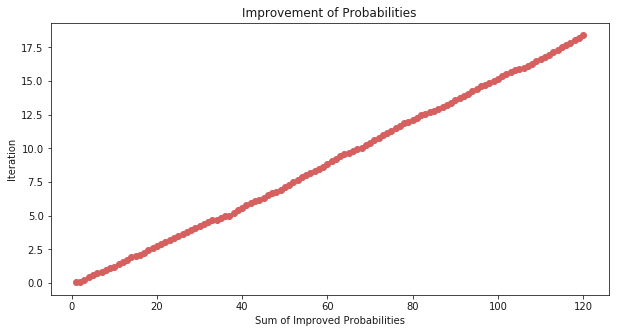#### 11.3 Validation¶

validate_heuristic_results(clf, to_optimize_v2, baseline, percentage=30, iterations=1000)

Baseline: 	200